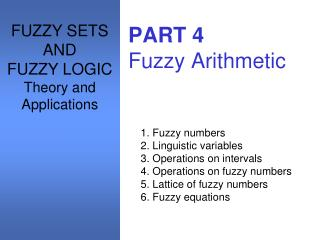Download PresentationPART 4 Fuzzy Arithmetic

Loading in 2 Seconds...

# PART 4 Fuzzy Arithmetic - PowerPoint PPT Presentation

FUZZY SETS AND FUZZY LOGIC Theory and Applications. PART 4 Fuzzy Arithmetic. 1. Fuzzy numbers 2. Linguistic variables 3. Operations on intervals 4. Operations on fuzzy numbers 5. Lattice of fuzzy numbers 6. Fuzzy equations. Fuzzy numbers. Three propertiesI am the owner, or an agent authorized to act on behalf of the owner, of the copyrighted work described.
Download Presentation## PART 4 Fuzzy Arithmetic

An Image/Link below is provided (as is) to download presentation

Download Policy: Content on the Website is provided to you AS IS for your information and personal use and may not be sold / licensed / shared on other websites without getting consent from its author.While downloading, if for some reason you are not able to download a presentation, the publisher may have deleted the file from their server.

- - - - - - - - - - - - - - - - - - - - - - - - - - E N D - - - - - - - - - - - - - - - - - - - - - - - - - -
Presentation Transcript
1. FUZZY SETS AND FUZZY LOGIC Theory and Applications PART 4Fuzzy Arithmetic 1. Fuzzy numbers 2. Linguistic variables 3. Operations on intervals 4. Operations on fuzzy numbers 5. Lattice of fuzzy numbers 6. Fuzzy equations

2. Fuzzy numbers • Three properties • A must be a normal fuzzy set; • αA must be a closed interval for every • the support of A, 0+A, must be bounded. A is a fuzzy set on R.

3. Fuzzy numbers

4. Fuzzy numbers • Theorem 4.1 Let Then, A is a fuzzy number if and only if there exists a closed interval such that

5. Fuzzy numbers • Theorem 4.1 (cont.) where is a function from that is monotonic increasing, continuous from the right, and such that ; is a function from that is monotonic decreasing, continuous from the left, and such that

6. Fuzzy numbers

7. Fuzzy numbers

8. Fuzzy numbers • Fuzzy cardinality Given a fuzzy set A defined on a finite universal set X, its fuzzy cardinality, , is a fuzzy number defined on N by the formula for all

9. Linguistic variables • The concept of a fuzzy number plays a fundamental role in formulatingquantitative fuzzyvariables. • Thefuzzy numbers represent linguistic concepts,such as very small, small, medium, and so on,as interpreted in a particular context, the resulting constructs are usually called linguisticvariables.

10. Linguistic variables • base variable Each linguistic variable the states of which are expressed by linguistic terms interpretedas specific fuzzy numbers is defined in terms of a base variable, the values of which arereal numbers within a specific range. A base variable is a variable in the classical sense,exemplified by any physical variable (e.g., temperature, etc.) as well as any other numerical variable, (e.g., age, probability, etc.).

11. Linguistic variables • Each linguistic variable is fully characterized by a quintuple (v, T, X, g, m). • v : the name of the variable. • T : the set of linguistic terms of v that refer to a base variable whose values range over a universal set X. • g:a syntactic rule (a grammar) for generating linguistic terms. • m : a semantic rule that assigns to each linguistic term t T.

12. Linguistic variables

13. Operations on intervals • Let ＊ denote any of the four arithmetic operations on closed intervals: addition ＋, subtraction —, multiplication • , and division /. Then,

14. Operations on intervals • Properties Let

15. Operations on intervals

16. Operations on fuzzy numbers • First method Let A and B denote fuzzy numbers. ＊ denote any of the four basic arithmetic operations. for any Since is a closed interval for each and A, B are fuzzy numbers, is also a fuzzy number.

17. Operations on fuzzy numbers • Second method

18. Operations on fuzzy numbers

19. Operations on fuzzy numbers

20. Operations on fuzzy numbers • Theorem 4.2 Let ＊ {＋, －, •, / }, and let A, B denote continuous fuzzy numbers. Then, the fuzzy set A＊B defined by is a continuous fuzzy number.

21. Lattice of fuzzy numbers • MIN and MAX

22. Lattice of fuzzy numbers

23. Lattice of fuzzy numbers

24. Lattice of fuzzy numbers • Theorem 4.3 LetMIN and MAXbe binary operations on R. Then, for any , the following properties hold:

25. Lattice of fuzzy numbers

26. Lattice of fuzzy numbers • Lattice It also can be expressed as the pair , where is a partial ordering defined as:

27. Lattice of fuzzy numbers

28. Fuzzy equations • A + X = B The difficulty of solving this fuzzy equation is caused by the fact that X = B－A is not thesolution. Let A = [a1,a2] and B = [b1,b2] be two closed intervals,which may be viewed as special fuzzy numbers.B－A = [b1－a2 ,b2 －a1], then

29. Fuzzy equations Let X = [x1, x2].

30. Fuzzy equations Let αA = [αa1, αa2],αB = [αb1,αb2], and αX = [αx1, αx2] for any .

31. Fuzzy equations • A．X = B A, B are fuzzy numbers on R+. It’s easy to show that X = B / A is not a solution of the equation.

32. Exercise 4 • 4.1 • 4.2 • 4.5 • 4.6 • 4.9